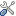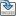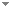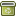# Programming relativity and gravity via a discrete pixel space in Planck level Simulation Hypothesis models

 Authors Abstract Outlined here is a simulation hypothesis approach that uses an expanding (the simulation clock-rate measured in units of Planck time) 4-axis hyper-sphere and mathematical particles that oscillate between an electric wave-state and a mass (unit of Planck mass per unit of Planck time) point-state. Particles are assigned a spin axis which determines the direction in which they are pulled by this (hyper-sphere pilot wave) expansion, thus all particles travel at, and only at, the velocity of expansion (the origin of $c$), however only the particle point-state has definable co-ordinates within the hyper-sphere. Photons are the mechanism of information exchange, as they lack a mass state they can only travel laterally (in hypersphere co-ordinate terms) between particles and so this hypersphere expansion cannot be directly observed, relativity then becomes the mathematics of perspective translating between the absolute (hypersphere) and the relative motion (3D space) co-ordinate systems. A discrete pixel' lattice geometry is assigned as the gravitational space. Units of $\hbar c$ physically' link particles into orbital pairs. As these are direct particle to particle links, a gravitational force between macro objects is not required, the gravitational orbit as the sum of these individual orbiting pairs. A 14.6 billion year old hyper-sphere (the sum of Planck black-hole units) has similar parameters to the cosmic microwave background. The Casimir force is a measure of the background radiation density. Keywords cosmic microwave background  cosmological constant  black-hole universe  Hubble constant  CMB  radiation density  Casimir force  arrow of time  simulation universe  mathematical universe Categories (categorize this paper) OptionsEdit this recordMark as duplicateExport citationFind it on ScholarRequest removal from indexRevision history

PhilArchive copy

Setup an account with your affiliations in order to access resources via your University's proxy server
Configure custom proxy (use this if your affiliation does not provide a proxy)

## Citations of this work BETA

No citations found.